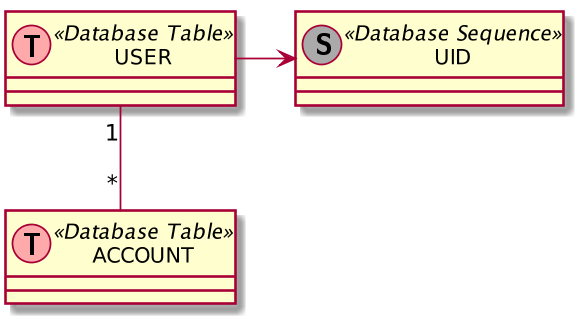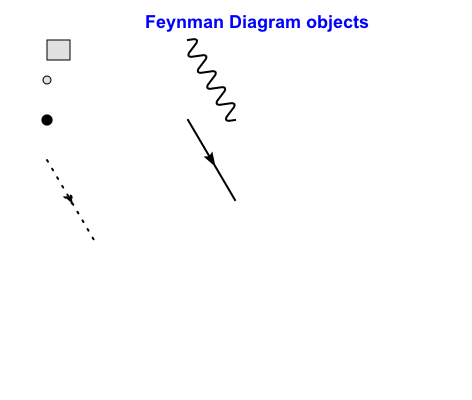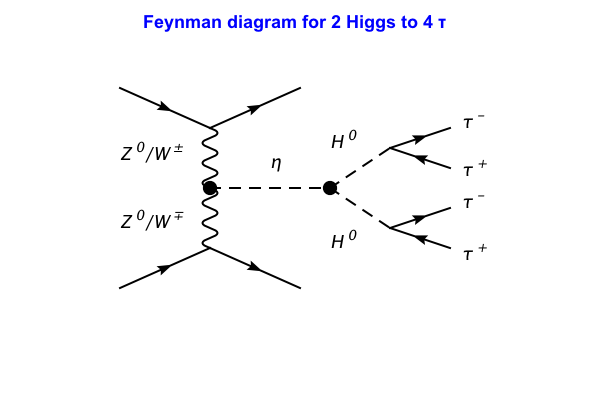# DMelt:Plots/Diagrams

Limitted access. First login to DataMelt if you are a full DataMelt member. Then login to HandWiki as a user.

## Drawing diagrams

A diagram is a symbolic representation of data according to some visualization technique. Read Diagram.

DMelt can use PlanUML language to create diagrams. See http://plantuml.com/ for the description. To create diagrams from a string or a text file, usejhplot.HDiagram class. This approach can be used to draw:

1. Sequence diagram
2. Usecase diagram
3. Class diagram
4. Activity diagram (here is the legacy syntax)
5. Component diagram
6. State diagram
7. Object diagram
8. Deployment diagram
9. Timing diagram

All such diagrams can be described in a text file, and then converted to a SVG, EPS, PDF, PNG images. Please read this manual (PDF) for plantuml syntax.

This small code that usesjhplot.HDiagram to create a high-quality image with the diagram:

creates the image shown here:## Drawing shapes programmically

Using DataMelt canvases, one can draw lines, arrows, ovals, interactive labels. One can use the same canvas as for plotting data and functions.

For example, use the canvasjhplot.HPlot. One can add objects using the method "add" and the call "update()" method to draw all objects at once:

from jhplot import *
from java.awt  import *
from jhplot.shapes  import *
c1=HPlot("Canvas")
c1.setGTitle("HShape package")
c1.setLegend(0)
c1.setRange(-4.0,4.0,0.0,20.0)
c1.visible()
arr=Arrow(0.85,0.5,0.85,0.7)
arr.setColor(Color.blue)
arr.setPosCoord("NDC")
lab=HLabel("Interactive label",-2,10);
c1.update()

## Drawing interactive diagrams

Let us show how to draw interactive diagrams using thejhplot.HPlotJa. This class can be used to show data, histograms and functions, but it is better suited for interactive diagram drawing. The class can be used to draw Feynman diagrams that are popular in particle physics. DataMelt can be used to draw Feynman diagrams programically using Java, Python (Jython) or any other scripting language supported by DataMelt.

Note that if you want to draw Feynman diagrams using a GUI, without programming, use JaxoDraw which was used in the past to createjhplot.HPlotJa class.

We will show below how to draw several elements of Feynman diagrams:

from java.awt import *
from jhplot import *

c1=HPlotJa("Canvas",600,400,1,1,0)
c1.setGTitle("Feynman Diagram objects", Color.blue)
c1.visible()
c1.showEditor(1)   # show diagram editor
gl=Diagram.Box(0.1,0.1) # box
gl.setRelWH(0.05,0.05,"NDC")
gl=Diagram.Blob(0.1,0.2) # blob
gl.setRelWH(0.01,0.01,"NDC")
gl=Diagram.Vertex(0, 0.1,0.3) # a vertex
gl.setRelWH(0.01,0.01,"NDC")
gl=Diagram.GLine(0.1,0.4)     # gluon line
gl.setStroke(2)
gl.setRelWH(0.1,0.2,"NDC")
gl=Diagram.PLine(0.4,0.1)     # gluon line
gl.setStroke(2)
gl.setRelWH(0.1,0.2,"NDC")
gl=Diagram.FLine(0.4,0.3)     # gluon line
gl.setStroke(2)
gl.setRelWH(0.1,0.2,"NDC")

c1.update()When define an object, we specify X and Y initial position using the NDC system (a system when X and Y sizes are between 0 and 1). The we define the extend in X and Y, relative to the position, using the method setRelWH(X,Y).

To learn about different elements of Feynman diagrams that can be shown in thejhplot.HPlotJa, look at thejhplot.jadraw.Diagram Java class. In addition, look at the packagejhplot/jadraw/package-summary which shows the API of objects to draw Feynman diagrams. To export to images, use "c1.export("image.pdf")" as for any canvas.

Let us draw a simple Feynman diagram for double Higgs production.

from java.awt import *
from jhplot import *

# position of the diagram on the canvas
Xpos=0.2
Ypos=0.22

c1=HPlotJa("Diagram",600,400,1,1,0)
c1.setGTitle("Feynman diagram for 2 Higgs to 4 &tau;", Color.blue)
c1.visible()
c1.showEditor(False)

# upper V shape
# start position x=0.05, y=0.1
gl=Diagram.FLine(Xpos,Ypos)
gl.setRelWH(0.15,0.1,"NDC")
gl.setStroke(2)

gl=Diagram.FLine(Xpos+0.15,Ypos)
gl.setRelWH(0.15,-0.1,"NDC")
gl.setStroke(2)

# lower V  shape
gl=Diagram.FLine(Xpos,Ypos+0.4)
gl.setRelWH(0.15,-0.1,"NDC")
gl.setStroke(2)

gl=Diagram.FLine(Xpos+0.15,Ypos+0.4)
gl.setRelWH(0.15,0.1,"NDC")
gl.setStroke(2)

# show blob at the center
gl=Diagram.Vertex(0, Xpos+0.15,Ypos+0.25)
gl.setRelWH(0.01,0.01,"NDC")

# vertical line like photon
gl=Diagram.PLine(Xpos+0.15,Ypos+0.1)
gl.setRelWH(0.0,0.3,"NDC")
gl.setStroke(2)

# horizontal dottedline for eta
gl=Diagram.SLine(Xpos+0.15,Ypos+0.25)
gl.setRelWH(0.2,0.0,"NDC")
gl.setArrow(False)
gl.setStroke(2)

# show blob at the end of horisonla line
gl=Diagram.Vertex(0, Xpos+0.35,Ypos+0.25)
gl.setRelWH(0.01,0.01,"NDC")

# show eta decay to Higgs
gl=Diagram.SLine(Xpos+0.35,Ypos+0.25)
gl.setRelWH(0.1,0.1,"NDC")
gl.setArrow(False);
gl.setStroke(2)

# show eta decay to Higgs
gl=Diagram.SLine(Xpos+0.35,Ypos+0.15)
gl.setRelWH(0.1,-0.1,"NDC")
gl.setArrow(False);
gl.setStroke(2)

# show upper higgs decay to tau
# upper fermion line
gl=Diagram.FLine(Xpos+0.45,Ypos+0.3)
gl.setRelWH(0.1,-0.05,"NDC")
gl.setFlip(False)
gl.setStroke(2)

# lower fermin line
gl=Diagram.FLine(Xpos+0.45,Ypos+0.35)
gl.setRelWH(-0.1,-0.05,"NDC")
gl.setStroke(2)

# show apper fermin line
gl=Diagram.FLine(Xpos+0.45,Ypos+0.1)
gl.setRelWH(0.1,-0.05,"NDC")
gl.setStroke(2)

# show lower higgs decay to tau
gl=Diagram.FLine(Xpos+0.45,Ypos+0.15)
gl.setRelWH(-0.1,-0.05,"NDC")
gl.setStroke(2)

# show text
gl=Diagram.Text("&eta;", Xpos+0.25, Ypos+0.2)

# show Higgs lines
gl=Diagram.Text("H^{ 0}", Xpos+0.35,Ypos+0.15)
gl=Diagram.Text("H^{ 0}", Xpos+0.35,Ypos+0.4)

# tau
t1=Diagram.Text("&tau;^{ +}", Xpos+0.57, Ypos+0.22)
t2=Diagram.Text("&tau;^{ -}", Xpos+0.57,Ypos+0.10)

# tau
t3=Diagram.Text("&tau;^{ -}", Xpos+0.57, Ypos+0.3)
t4=Diagram.Text("&tau;^{ +}", Xpos+0.57, Ypos+0.43)

# show text
gl=Diagram.Text("Z^{ 0}/W^{ &plusmn;}", Xpos,Ypos+0.18)

# show text
gl=Diagram.Text("Z^{ 0}/W^{ &#8723;}", Xpos, Ypos+0.35)
# c1.update()